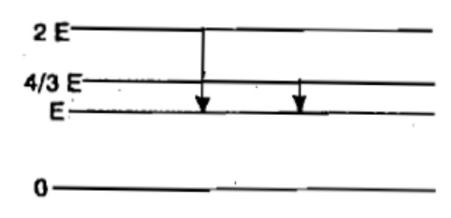Consider an electron in the nth orbit of a hydrogen atom in the Bohr model. The circumference of the orbit can be expressed in terms of the de-Broglie wavelength $\lambda$ of that electron as:

(1) (0.529) n$\lambda$           (2) $\sqrt{n\lambda }$             (3) (13.6) $\lambda$         (4) n$\lambda$

Concept Questions :-

Bohr's model of atom
High Yielding Test Series + Question Bank - NEET 2020

Difficulty Level:

In the Bohr's model of a hydrogen atom, the centripetal force is furnished by the Coulomb attraction between the proton and the electron.  If ${\alpha }_{0}$ is the radius of the ground state orbit, m is the mass and e is the charge on the electron, ${\epsilon }_{0}$ is the vaccum permittivity, the speed of the electron is  

(1) zero          (2) $\frac{e}{\sqrt{{\epsilon }_{0}{a}_{0}m}}$           (3) $\frac{e}{\sqrt{4{\mathrm{\pi \epsilon }}_{0}{\mathrm{a}}_{0}\mathrm{m}}}$               (4) $\frac{\sqrt{4{\mathrm{\pi \epsilon }}_{0}{\mathrm{a}}_{0}\mathrm{m}}}{e}$

Concept Questions :-

Bohr's model of atom
High Yielding Test Series + Question Bank - NEET 2020

Difficulty Level:

The ground state energy of H-atom is 13.6 eV. The energy needed to ionise H-atom from its second excited state 

(1) 1.51 eV          (2) 3.4 eV            (3) 13.6 eV             (4) 12.1 eV

Concept Questions :-

Bohr's model of atom
High Yielding Test Series + Question Bank - NEET 2020

Difficulty Level:

The element which has a ${k}_{a}$ x-rays line of wavelength 1.8 $\stackrel{0}{A}$ is

(1) Co, Z=27      (2) Iron, Z = 26        (3) Mn, Z = 25           (4) Ni, Z = 28

Concept Questions :-

Spectral series
High Yielding Test Series + Question Bank - NEET 2020

Difficulty Level:

The given diagram indicates the energy levels of a certain atom. When the system moves from 2E level to E, a photon of wavelength $\lambda$ is emitted. The wavelength of photon produced during its transition from $\frac{4E}{3}$ level to E is(1) $\frac{\lambda }{3}$           (2) $\frac{3\lambda }{4}$        (3) $\frac{4\lambda }{3}$            (4) $3\lambda$

Concept Questions :-

Spectral series
High Yielding Test Series + Question Bank - NEET 2020

Difficulty Level:

The wavelength of the first spectral line in the Balmer series of hydrogen atom is 6561 $\stackrel{0}{A}$. The wavelength of the second spectral line in the Balmer series of singly ionized helium atom is [IIT-JEE 2011]

(1) 1215 $\stackrel{0}{A}$        (2) 1640 $\stackrel{0}{A}$            (3) 2430 $\stackrel{0}{A}$           (4) 4687 $\stackrel{0}{A}$

Concept Questions :-

Spectral series
High Yielding Test Series + Question Bank - NEET 2020

Difficulty Level:

Energy E of a hydrogen atom with principal quantum number n is given by $E=\frac{-13.6}{{n}^{2}}eV$.  The energy of a photon ejected when the electron jumps from n = 3 state to n = 2 state of hydrogen, is approximately 

(1) 1.5 eV         (2) 0.85 eV          (3) 3.4 eV            (4) 1.9 eV

Concept Questions :-

Bohr's model of atom
High Yielding Test Series + Question Bank - NEET 2020

Difficulty Level:

The energy of ground electronic state of hydrogen atom is -13.6 eV.  The energy of the first excited state will be 

(1) -54.4 eV         (2) -27.2 eV           (3) -6.8 eV               (4) -3.4 eV

Concept Questions :-

Bohr's model of atom
High Yielding Test Series + Question Bank - NEET 2020

Difficulty Level:

The total energy of an electron in the first excited state of hydrogen is about -3.4 eV. Its kinetic energy in this state is 

(1) -3.4 eV            (2) -6.8 eV             (3) 6.8 eV           (4) 3.4 eV

Concept Questions :-

Bohr's model of atom
High Yielding Test Series + Question Bank - NEET 2020

Difficulty Level:

The energy of an electron in excited hydrogen atom is -3.4 eV. Then according to Bohr's theory, the angular momentum of the electron in Js is [Kerala PET 2002]

(1)

(2)

(3)

(4)

Concept Questions :-

Bohr's model of atom